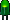• Home• Patreon• Links• Books
Number Converting:

(article continued from previous page)

Converting from hexadecimal to binary is as easy as converting from binary to hexadecimal. Simply look up each hexadecimal digit to obtain the equivalent group of four binary digits.

 Hexadecimal: 0 1 2 3 4 5 6 7 Binary: 0000 0001 0010 0011 0100 0101 0110 0111
 Hexadecimal: 8 9 A B C D E F Binary: 1000 1001 1010 1011 1100 1101 1110 1111

 Hexadecimal = A 2 D E Binary = 1010 0010 1101 1110 = 1010001011011110 binary

When converting from hexadecimal to octal, it is often easier to first convert the hexadecimal number into binary and then from binary into octal. For example, to convert A2DE hex into octal:

(from the previous example)

 Hexadecimal = A 2 D E Binary = 1010 0010 1101 1110 = 1010001011011110 binary

Binary: 1010001011011110 = 001 010 001 011 011 110

Then, look up each group in a table:

 Binary: 000 001 010 011 100 101 110 111 Octal: 0 1 2 3 4 5 6 7

 Binary = 001 010 001 011 011 110 Octal = 1 2 1 3 3 6 = 121336 octal

Therefore, through a two-step conversion process, hexadecimal A2DE equals binary 1010001011011110 equals octal 121336.